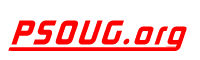Quick Search: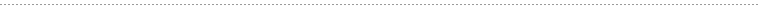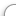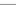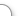CODE Oracle PL/SQL Code Library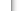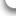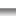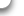JOBS Find Or Post Oracle JobsFORUM Oracle Discussion & ChatPHP Code: Build Date Select Boxes Jump to: Select a Location PSOUG Home Page Oracle Code Library mySQL Code Library PHP Code Library JavaScript Code Library Oracle Terms & Definitions Oracle Error Codes PSOUG Community Blogs Oracle Jobs Board PSOUG Forum Oracle User Group Directory Free Oracle Magazines Online Learning Center PSOUG Presentations Advanced Code Search News and Events Sponsors Page Submit Code Contact UsSnippet Name: Build Date Select Boxes

Description: A function that builds select boxes for the month,day,and year. It will default to mmddyyyy, current date, or specified date depending on the parameters.

Comment: (none)

Language: PHP
Highlight Mode: PHP

```<?PHP

FUNCTION buildDate(\$name, \$m, \$d, \$y) {
\$date = DATE("m-d-Y",STRTOTIME("now"));
\$date_array = EXPLODE("-",\$date);
\$now_m = "";
\$now_d = "";
\$now_y = "";
\$month = '<select class="small" name="'.\$name.'_month">';
\$month .= '<option value="" selected disabled>mm</option>';
FOR (\$i=1;\$i<=12;\$i++) {
IF ( \$m != "" ) {
IF ( \$m == \$i ) {
\$now_m = "selected";
}
}ELSEIF ( \$m == "0" ) {
\$now_m = "";
}ELSE{
IF ( \$date_array == \$i ) {
\$now_m = "selected";
}
}
\$month .= '<option value="'.\$i.'" '.\$now_m.'>'.\$i.'</option>';
\$now_m = "";
}//END month for loop
\$month .= '</select>';
\$day = '<select class="small" name="'.\$name.'_day">';
\$day .= '<option value="" selected disabled>dd</option>';
FOR (\$i=1;\$i<=31;\$i++) {
IF ( \$d != "" ) {
IF ( \$d == \$i ) {
\$now_d = "selected";
}
}ELSEIF ( \$d == "0" ) {
\$now_d = "";
}ELSEIF ( \$d == \$i ) {
\$now_d = "selected";
}ELSE{
IF ( \$date_array == \$i ) {
\$now_d = "selected";
}
}
\$day .= '<option value="'.\$i.'" '.\$now_d.'>'.\$i.'</option>';
\$now_d = "";
}//END day for loop
\$day .= '</select>';
\$year = '<select class="small" name="'.\$name.'_year">';
\$year .= '<option value="" selected disabled>yyyy</option>';
FOR (\$i=GMDATE("Y"); \$i <=SUBSTR(get330Date(),0,4); \$i++) {
IF ( \$y != "" ) {
IF ( \$y == \$i ) {
\$now_y = "selected";
}
}ELSEIF ( \$y == "0" ) {
\$now_y = "";
}ELSEIF ( \$y == \$i ) {
\$now_y = "selected";
}ELSE{
IF ( \$date_array == \$i ) {
\$now_y = "selected";
}
}
\$year .= '<option value="'.\$i.'" '.\$now_y.'>'.\$i.'</option>';
\$now_y = "";
}//END year for loop
\$year .= '</select>';
//-------------------------------------------
ECHO \$month." ".\$day." ".\$year;
}//END buildDate function
// ------------------------------------
FUNCTION get330Date() {
RETURN DATE("Y-m-d", MKTIME(0, 0, 0, SUBSTR(GMDATE("Y m d"),5,2),
SUBSTR(GMDATE("Y m d"),8,2) + 330,
SUBSTR(GMDATE("Y m d"),0,4)))."<br />";
}

?>```FreeOracle MagazineSubscriptionsand Oracle White Papers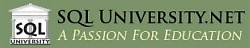SQL University.net courses meet the most demanding needs of the business world for advanced education in a cost-effective manner. SQL University.net courses are available immediately for IT professionals and can be taken without disruption of your workplace schedule or processes.

Compared to traditional travel-based training, SQL University.net saves time and valuable corporate resources, allowing companies to do more with less. That's our mission, and that's what we deliver.